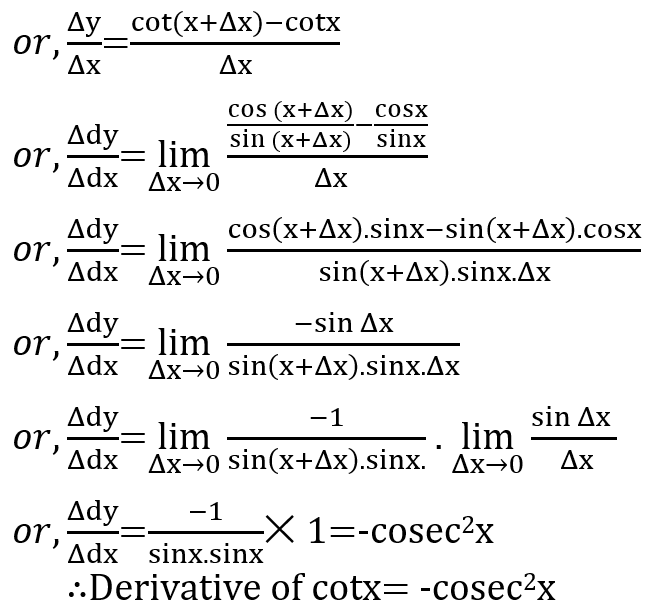# Derivative of trigonometric function tanx and cotxDERIVATE OF tanx
Let y=tanx……. iThen,Now,
Subtracting i from ii we get,By the first principle,DERIVATE OF cotx
Let y=cotx……. iThen,Now,
Subtracting i from ii we get,By the first principle,